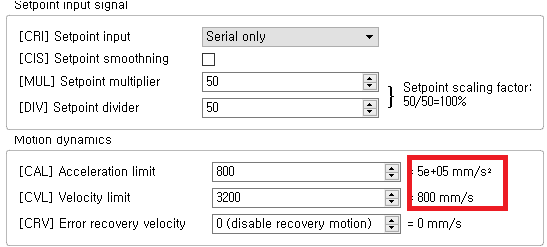# CAL, CVL, CRV Calculation method

Hi. We are using IONI 4X.

We use position mode and want to control speed and acceleration.
At this time, we want changed the CAL, CVL, and so on.
At this time, we want to know how the values of the parameters we entered and the values calculated are calculated.
What we would like to know is how to calculate the actual mm / s figures to MUL, DIV, CAL CVLMy hardware configuration:

• BLDC Motor
• 1.6.0
• IONI 4X

Hi! You can find the calculation formulas here:

https://granitedevices.com/wiki/Signal_path_of_motor_drive

I hope this helps!